# CS229编程7：K-means聚类与主成分分析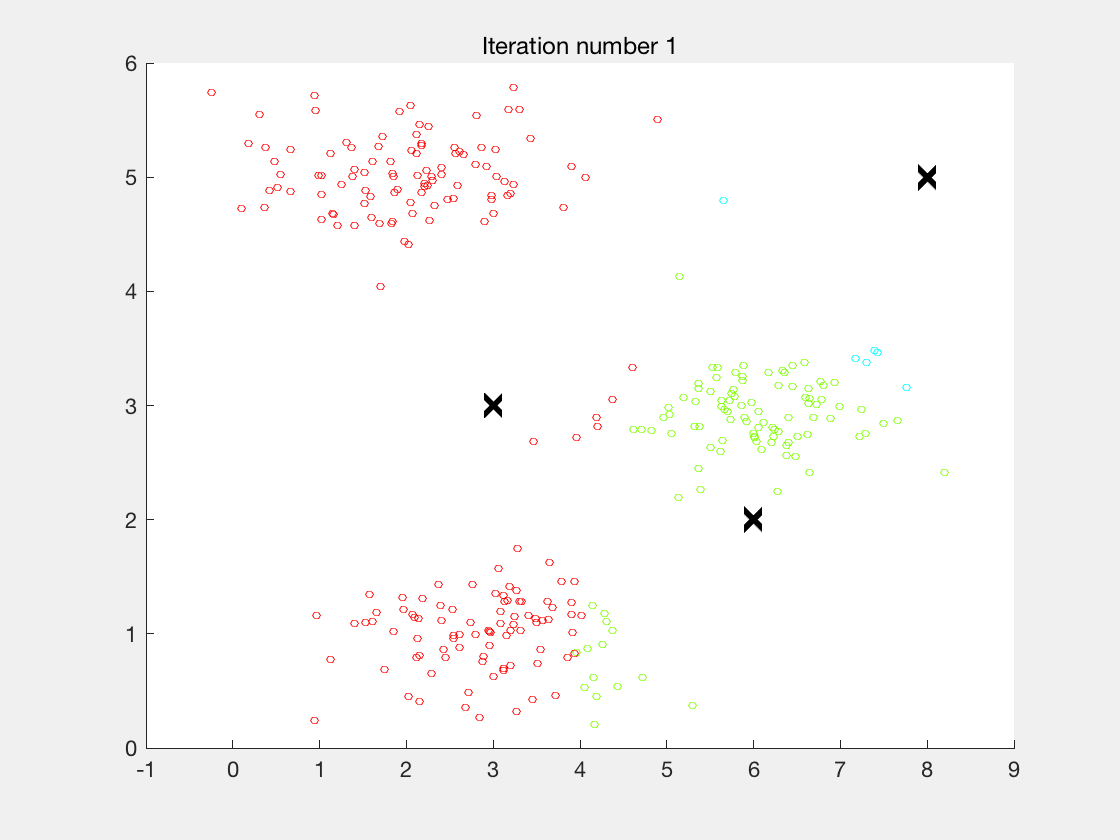斯坦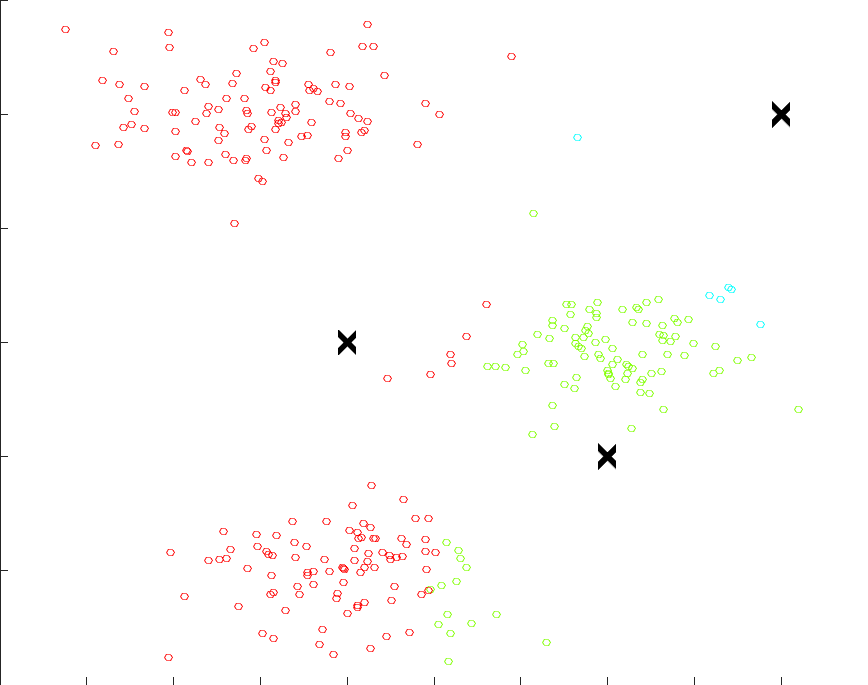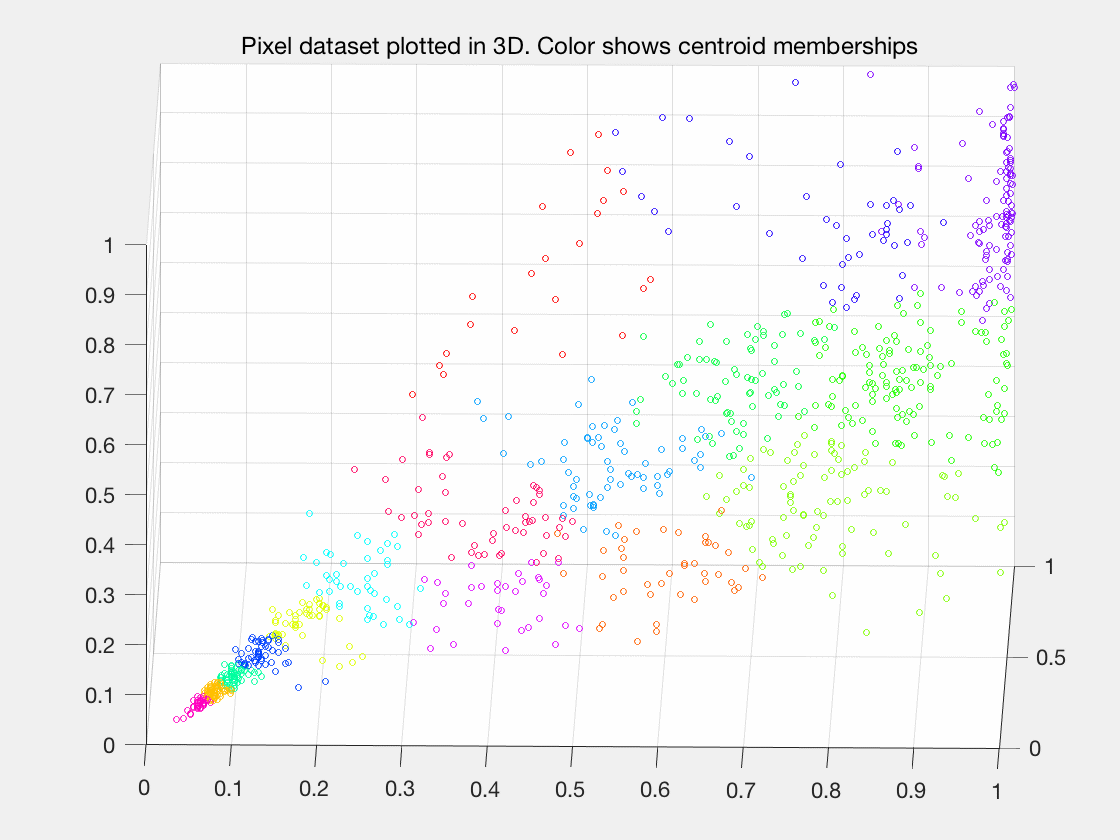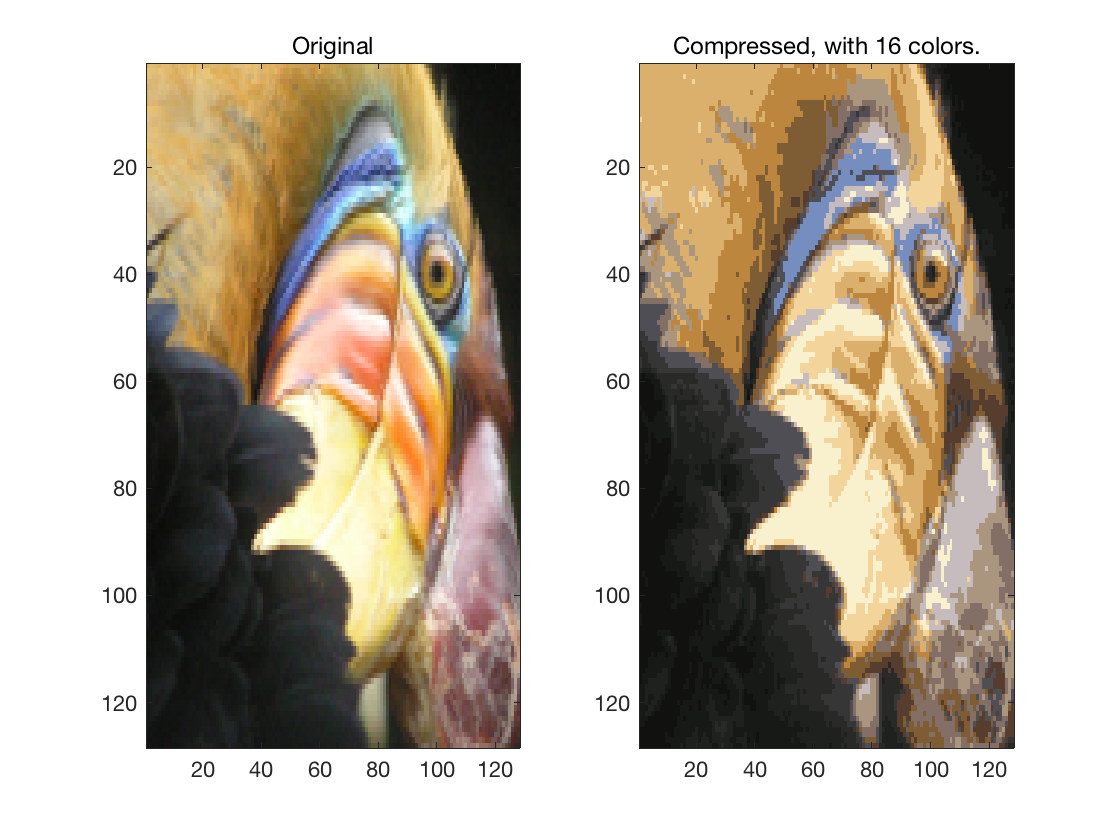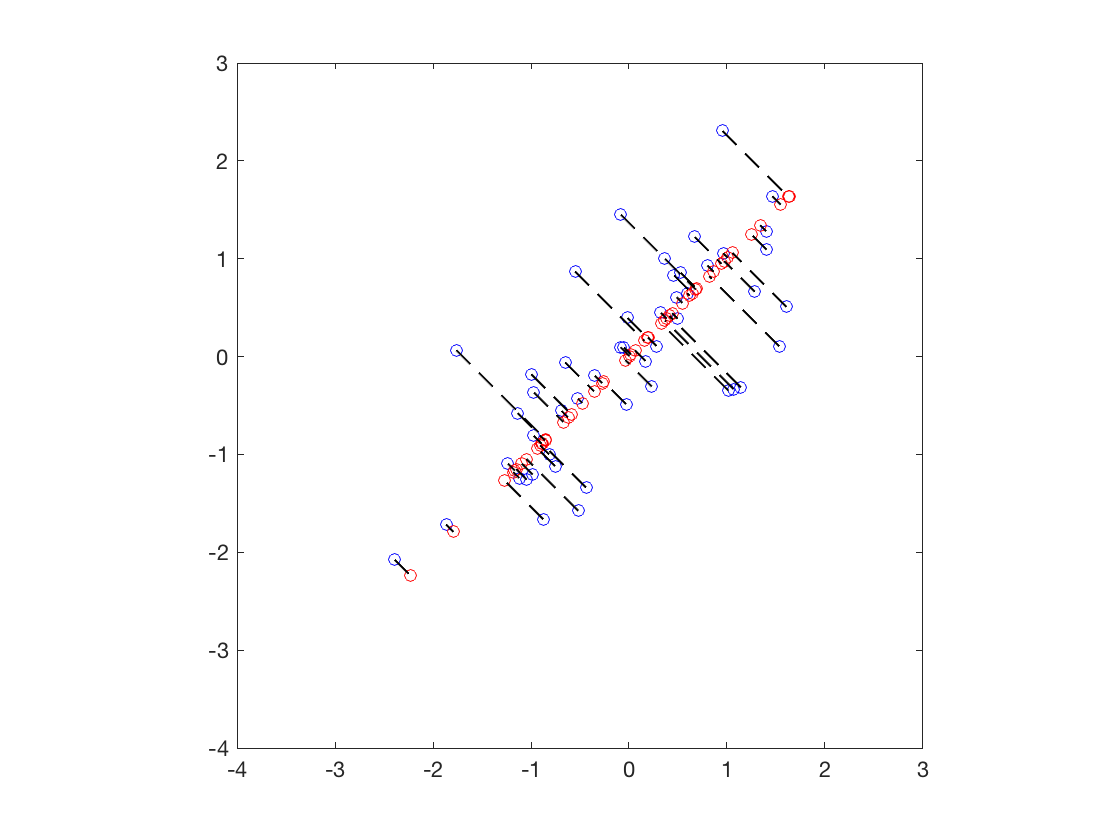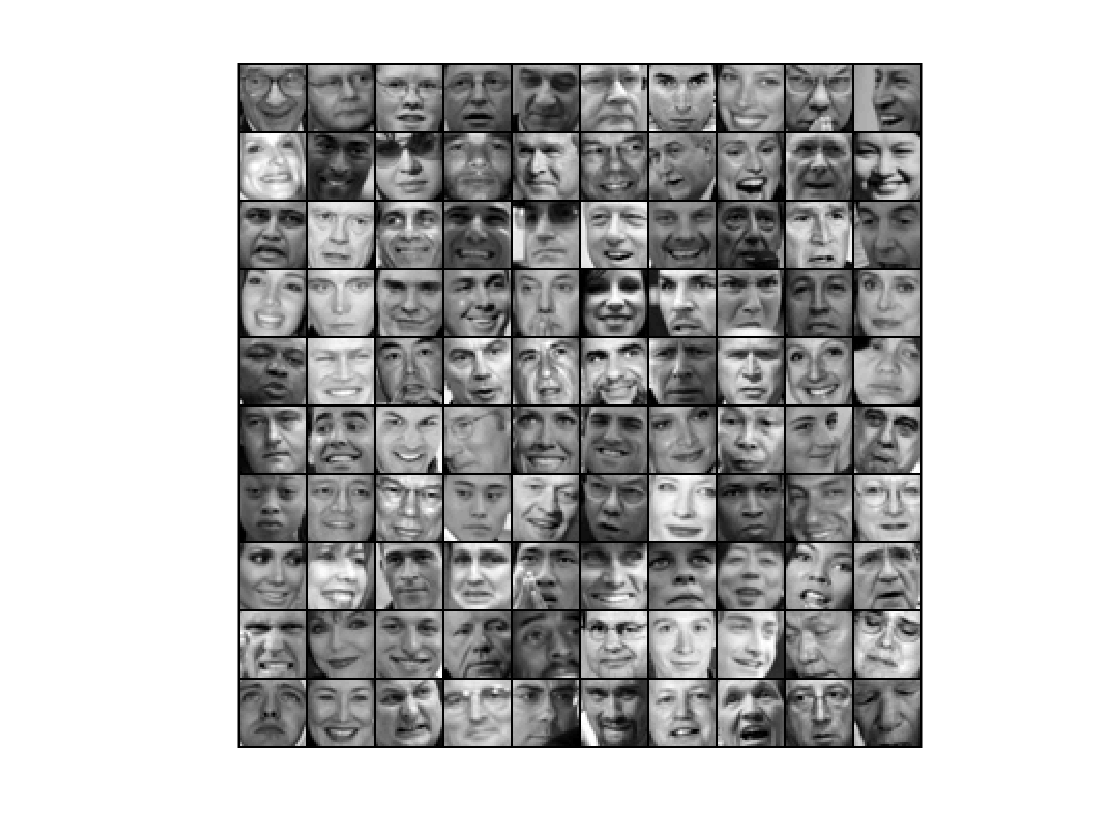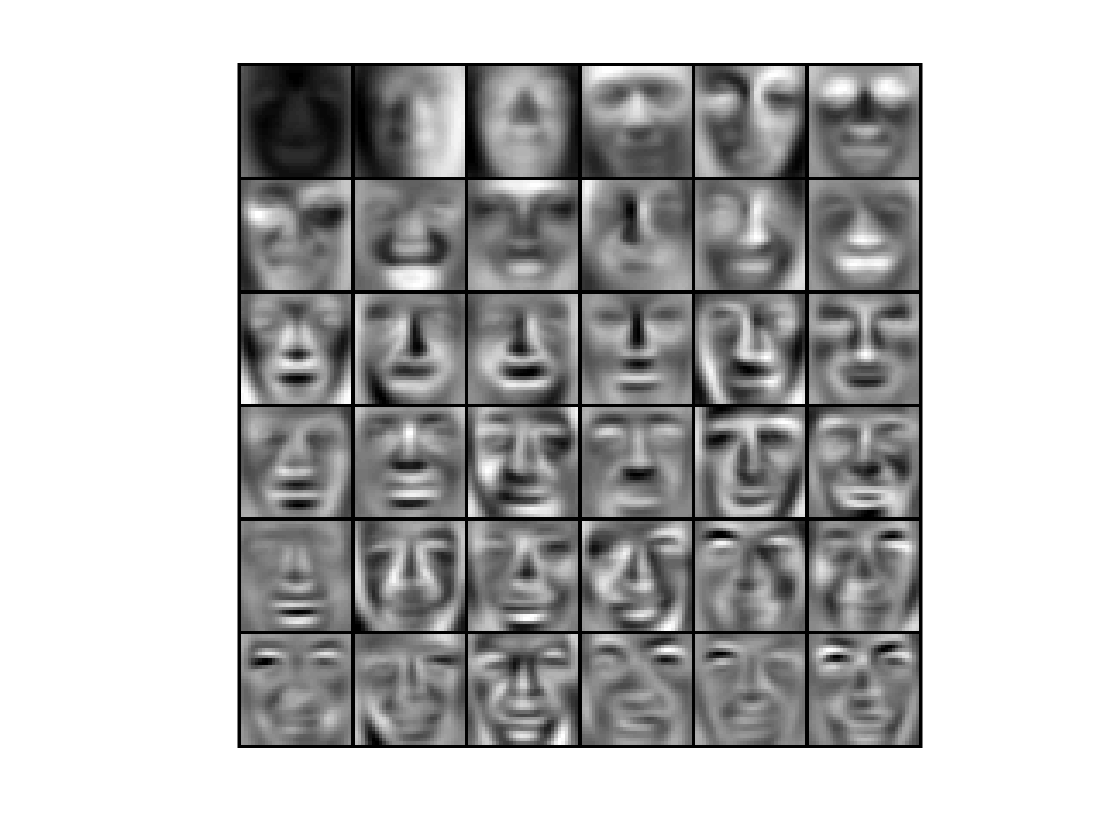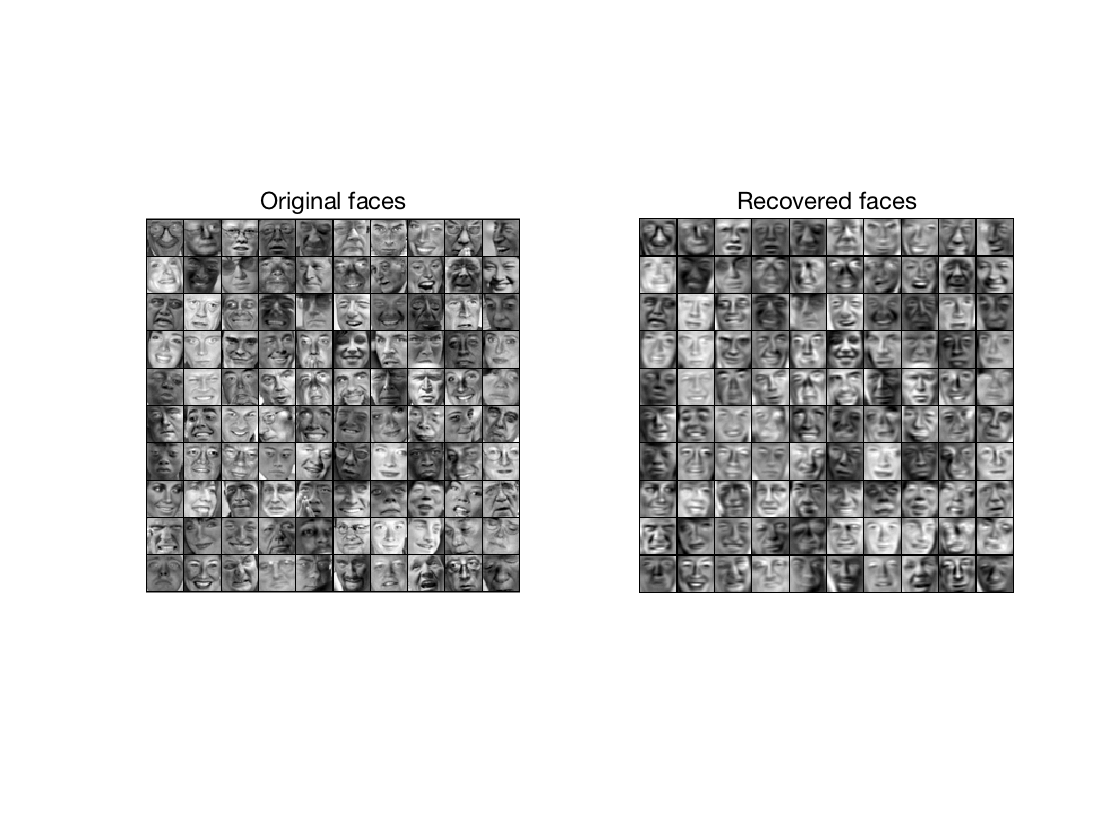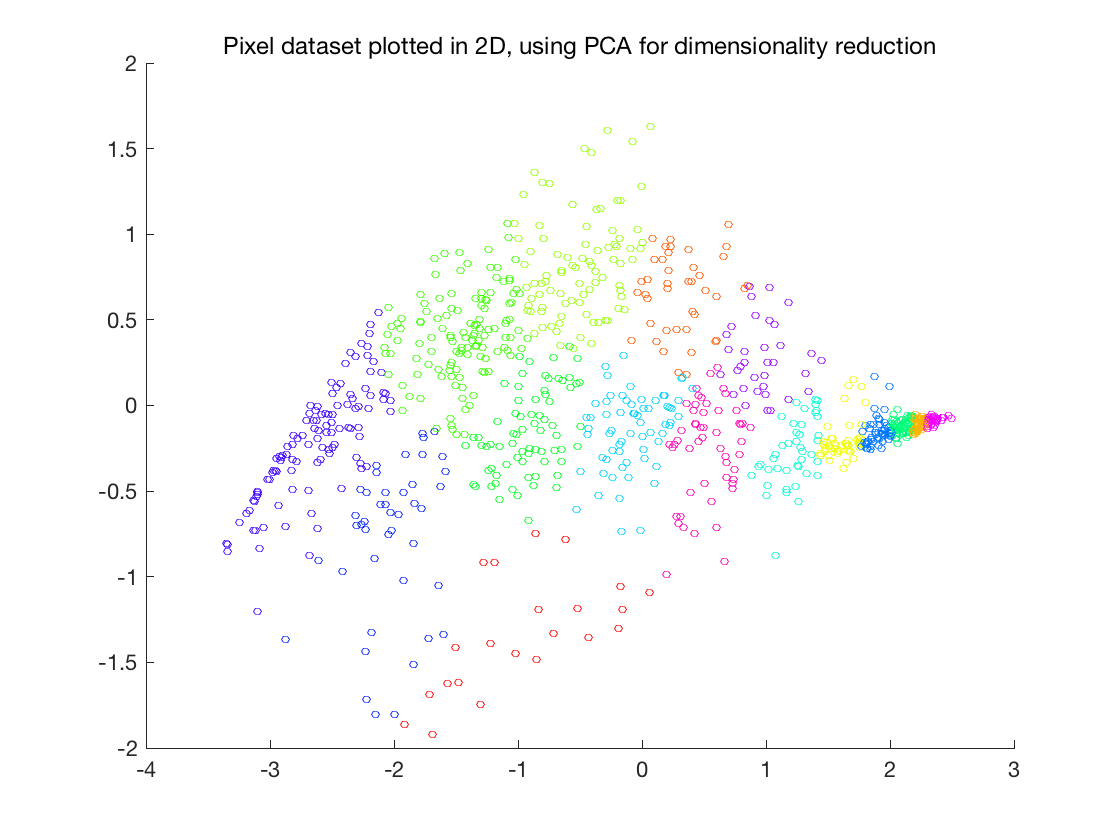福ML（Matlab）公开课，这次练习先实现K-means聚类算法并应用于图像压缩，然后实现PCA并用于人脸图像，最后展示高维数据的可视化技巧。我发现matlab做动画特别方便，顺手把算法执行过程动画化了。

## K-means聚类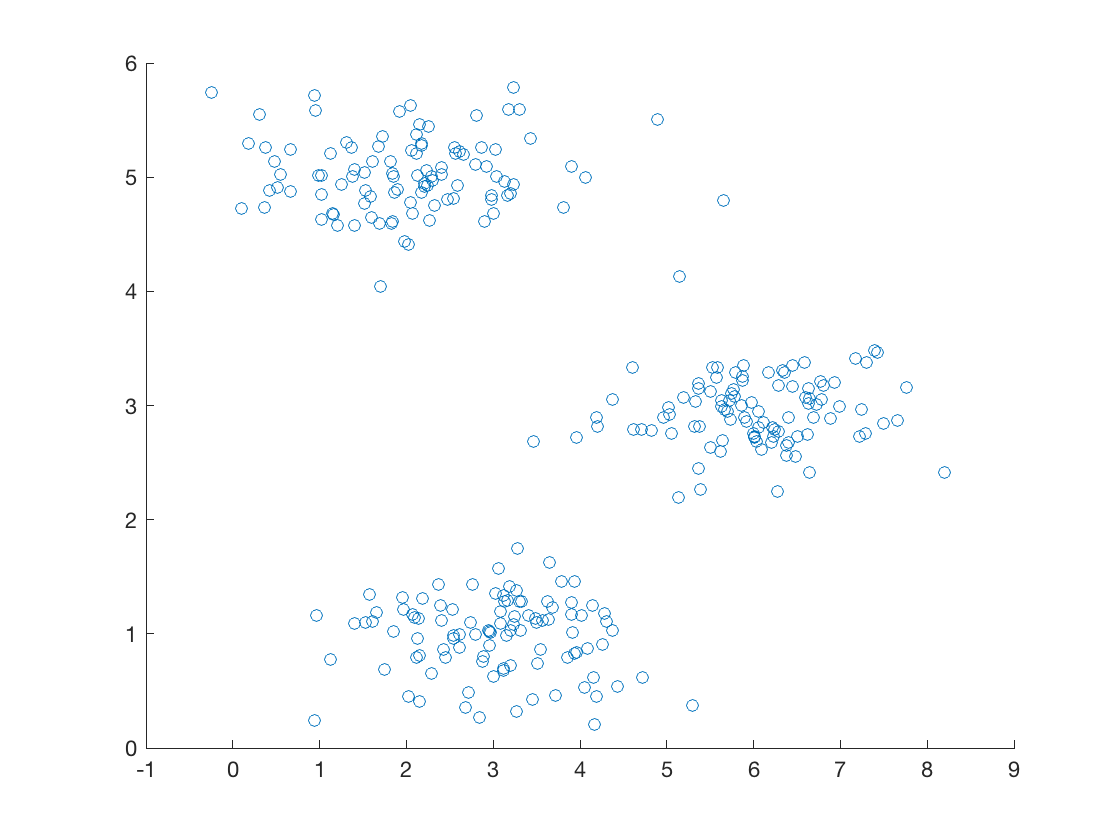```% Initialize centroids
centroids = kMeansInitCentroids(X, K);

for iter = 1:iterations

% Cluster assignment step: Assign each data point to the
% closest centroid. idx(i) corresponds to cˆ(i), the index
% of the centroid assigned to example i
idx = findClosestCentroids(X, centroids);

% Move centroid step: Compute means based on centroid
% assignments
centroids = computeMeans(X, idx, K);

end```

1. 将每个数据点分配给最近的重心

2. 分配导致重心变化，于是重新计算重心

K-means最终一定会收敛，但视初始化的重心不同，最终得到的聚类结果不一定是最优的。所以实践的时候一般初始化不同的重心，然后选择损失函数最小的聚类结果。

### 随机初始化

```function centroids = kMeansInitCentroids(X, K)
%KMEANSINITCENTROIDS This function initializes K centroids that are to be
%used in K-Means on the dataset X
%   centroids = KMEANSINITCENTROIDS(X, K) returns K initial centroids to be
%   used with the K-Means on the dataset X
%

% You should return this values correctly
centroids = zeros(K, size(X, 2));

% ====================== YOUR CODE HERE ======================
% Instructions: You should set centroids to randomly chosen examples from
%               the dataset X
%

% Initialize the centroids to be random examples
% Randomly reorder the indices of examples
randidx = randperm(size(X, 1));
% Take the first K examples as centroids
centroids = X(randidx(1:K), :);

% =============================================================

end```

### 寻找最近重心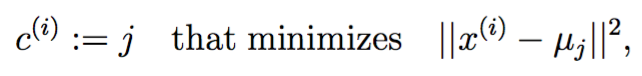```function idx = findClosestCentroids(X, centroids)
%FINDCLOSESTCENTROIDS computes the centroid memberships for every example
%   idx = FINDCLOSESTCENTROIDS (X, centroids) returns the closest centroids
%   in idx for a dataset X where each row is a single example. idx = m x 1
%   vector of centroid assignments (i.e. each entry in range [1..K])
%

% Set K
K = size(centroids, 1);

% You need to return the following variables correctly.
idx = zeros(size(X,1), 1);

% ====================== YOUR CODE HERE ======================
% Instructions: Go over every example, find its closest centroid, and store
%               the index inside idx at the appropriate location.
%               Concretely, idx(i) should contain the index of the centroid
%               closest to example i. Hence, it should be a value in the
%               range 1..K
%
% Note: You can use a for-loop over the examples to compute this.
%
for i = 1:size(X,1)
means = 0;
for k = 1:size(X,2)
means = means + (X(i,k) - centroids(1,k))^2;
end
id = 1;
for j = 2: K
temp = 0;
for k = 1:size(X,2)
temp = temp + (X(i,k) - centroids(j,k))^2;
end
if temp <= means
means = temp;
id = j;
end
end
idx(i) = id;
end

% =============================================================

end```

```   for k = 1:size(X,2)
means = means + (X(i,k) - centroids(1,k))^2;
end```

```%% ================= Part 1: Find Closest Centroids ====================
%  To help you implement K-Means, we have divided the learning algorithm
%  into two functions -- findClosestCentroids and computeCentroids. In this
%  part, you shoudl complete the code in the findClosestCentroids function.
%
fprintf('Finding closest centroids.\n\n');

% Load an example dataset that we will be using

% Select an initial set of centroids
K = 3; % 3 Centroids
initial_centroids = [3 3; 6 2; 8 5];

% Find the closest centroids for the examples using the
% initial_centroids
idx = findClosestCentroids(X, initial_centroids);

fprintf('Closest centroids for the first 3 examples: \n')
fprintf(' %d', idx(1:3));
fprintf('\n(the closest centroids should be 1, 3, 2 respectively)\n');

fprintf('Program paused. Press enter to continue.\n');
pause;```

### 计算重心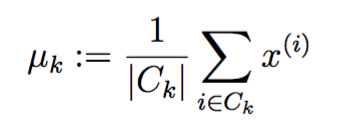```function centroids = computeCentroids(X, idx, K)
%COMPUTECENTROIDS returs the new centroids by computing the means of the
%data points assigned to each centroid.
%   centroids = COMPUTECENTROIDS(X, idx, K) returns the new centroids by
%   computing the means of the data points assigned to each centroid. It is
%   given a dataset X where each row is a single data point, a vector
%   idx of centroid assignments (i.e. each entry in range [1..K]) for each
%   example, and K, the number of centroids. You should return a matrix
%   centroids, where each row of centroids is the mean of the data points
%   assigned to it.
%

% Useful variables
[m n] = size(X);

% You need to return the following variables correctly.
centroids = zeros(K, n);

% ====================== YOUR CODE HERE ======================
% Instructions: Go over every centroid and compute mean of all points that
%               belong to it. Concretely, the row vector centroids(i, 🙂
%               should contain the mean of the data points assigned to
%               centroid i.
%
% Note: You can use a for-loop over the centroids to compute this.
%

for i = 1:K
Ck = 0;
for j = 1:m
if idx(j) == i;
Ck = Ck + 1;
for k = 1:n
centroids(i,k) = centroids(i,k) + X(j,k);
end
end
end
for k = 1:n
centroids(i,k) = centroids(i,k) / Ck;
end
end

% =============================================================

end```

### 运行K-means

```function [centroids, idx] = runkMeans(X, initial_centroids, ...
max_iters, plot_progress)
%RUNKMEANS runs the K-Means algorithm on data matrix X, where each row of X
%is a single example
%   [centroids, idx] = RUNKMEANS(X, initial_centroids, max_iters, ...
%   plot_progress) runs the K-Means algorithm on data matrix X, where each
%   row of X is a single example. It uses initial_centroids used as the
%   initial centroids. max_iters specifies the total number of interactions
%   of K-Means to execute. plot_progress is a true/false flag that
%   indicates if the function should also plot its progress as the
%   learning happens. This is set to false by default. runkMeans returns
%   centroids, a Kxn matrix of the computed centroids and idx, a m x 1
%   vector of centroid assignments (i.e. each entry in range [1..K])
%

% Set default value for plot progress
if ~exist('plot_progress', 'var') || isempty(plot_progress)
plot_progress = false;
end

% Plot the data if we are plotting progress
if plot_progress
figure;
hold on;
end

% Initialize values
[m n] = size(X);
K = size(initial_centroids, 1);
centroids = initial_centroids;
previous_centroids = centroids;
idx = zeros(m, 1);

% Run K-Means
for i=1:max_iters

% Output progress
fprintf('K-Means iteration %d/%d...\n', i, max_iters);
if exist('OCTAVE_VERSION')
fflush(stdout);
end

% For each example in X, assign it to the closest centroid
idx = findClosestCentroids(X, centroids);

% Optionally, plot progress here
if plot_progress
plotProgresskMeans(X, centroids, previous_centroids, idx, K, i);
previous_centroids = centroids;

frame=getframe(gcf);
im=frame2im(frame);
[I,map]=rgb2ind(im,256);
if i==1
imwrite(I,map,'ex.gif','gif','Loopcount',Inf,'DelayTime',0.2);
else
imwrite(I,map,'ex.gif','gif','WriteMode','append','DelayTime',0.2);
end
end

% Given the memberships, compute new centroids
centroids = computeCentroids(X, idx, K);
end

% Hold off if we are plotting progress
if plot_progress
hold off;
end

end```

```        frame=getframe(gcf);
im=frame2im(frame);
[I,map]=rgb2ind(im,256);
if i==1
imwrite(I,map,'ex.gif','gif','Loopcount',Inf,'DelayTime',0.2);
else
imwrite(I,map,'ex.gif','gif','WriteMode','append','DelayTime',0.2);
end```## 图像压缩```%% ============= Part 4: K-Means Clustering on Pixels ===============
%  In this exercise, you will use K-Means to compress an image. To do this,
%  you will first run K-Means on the colors of the pixels in the image and
%  then you will map each pixel on to it's closest centroid.
%
%  You should now complete the code in kMeansInitCentroids.m
%

fprintf('\nRunning K-Means clustering on pixels from an image.\n\n');

%  Load an image of a bird

% If imread does not work for you, you can try instead

A = A / 255; % Divide by 255 so that all values are in the range 0 - 1

% Size of the image
img_size = size(A);

% Reshape the image into an Nx3 matrix where N = number of pixels.
% Each row will contain the Red, Green and Blue pixel values
% This gives us our dataset matrix X that we will use K-Means on.
X = reshape(A, img_size(1) * img_size(2), 3);```

```% Run your K-Means algorithm on this data
% You should try different values of K and max_iters here
K = 16;
max_iters = 10;

% When using K-Means, it is important the initialize the centroids
% randomly.
% You should complete the code in kMeansInitCentroids.m before proceeding
initial_centroids = kMeansInitCentroids(X, K);

% Run K-Means
[centroids, idx] = runkMeans(X, initial_centroids, max_iters);

fprintf('Program paused. Press enter to continue.\n');
pause;```

idx就是每个像素点最近的重心的index，将像素点替换为重心就得到压缩后的图片：

```%% ================= Part 5: Image Compression ======================
%  In this part of the exercise, you will use the clusters of K-Means to
%  compress an image. To do this, we first find the closest clusters for
%  each example. After that, we

fprintf('\nApplying K-Means to compress an image.\n\n');

% Find closest cluster members
idx = findClosestCentroids(X, centroids);

% Essentially, now we have represented the image X as in terms of the
% indices in idx.

% We can now recover the image from the indices (idx) by mapping each pixel
% (specified by it's index in idx) to the centroid value
X_recovered = centroids(idx,:);

% Reshape the recovered image into proper dimensions
X_recovered = reshape(X_recovered, img_size(1), img_size(2), 3);

% Display the original image
subplot(1, 2, 1);
imagesc(A);
title('Original');

% Display compressed image side by side
subplot(1, 2, 2);
imagesc(X_recovered)
title(sprintf('Compressed, with %d colors.', K));

fprintf('Program paused. Press enter to continue.\n');
pause;```## 主成分分析

### 简单数据集

```%% ================== Part 1: Load Example Dataset  ===================
%  We start this exercise by using a small dataset that is easily to
%  visualize
%
fprintf('Visualizing example dataset for PCA.\n\n');

%  The following command loads the dataset. You should now have the
%  variable X in your environment

%  Visualize the example dataset
plot(X(:, 1), X(:, 2), 'bo');
axis([0.5 6.5 2 8]); axis square;

fprintf('Program paused. Press enter to continue.\n');
pause;```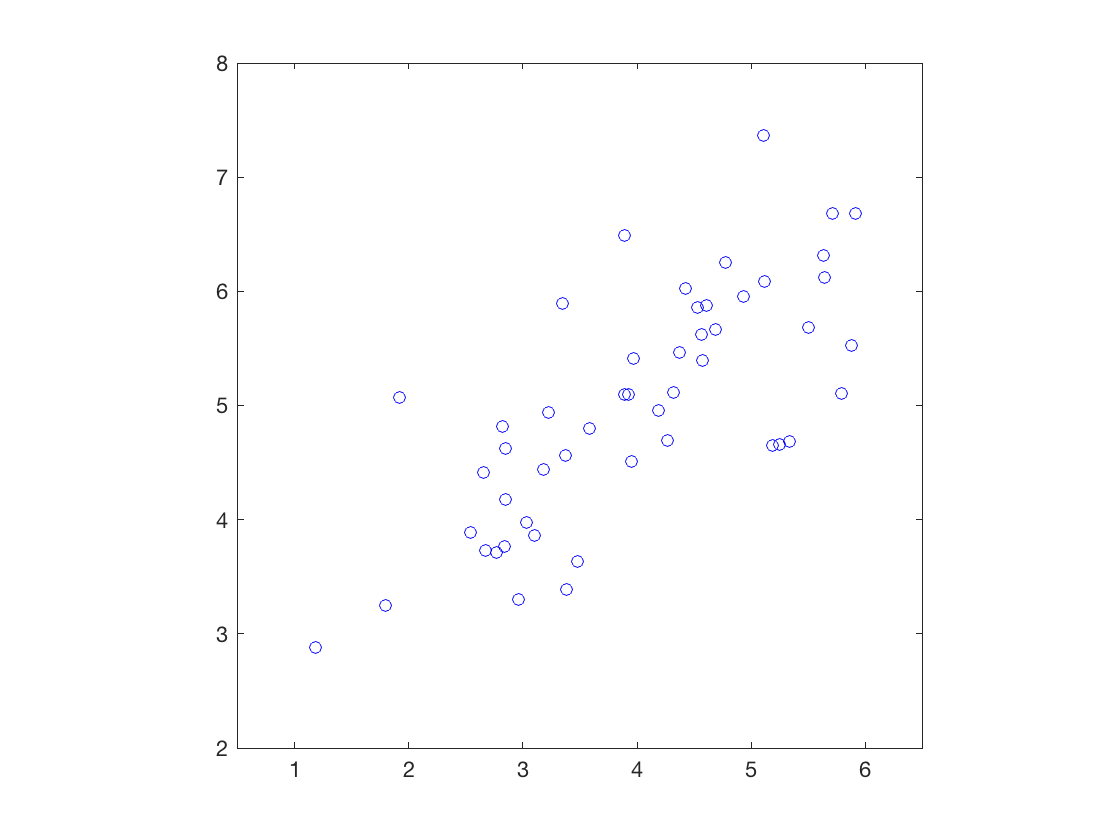### 实现PCA

1. 计算协方差矩阵

2. 利用matlab的SVD方法得到特征向量和特征值

### 数据标准化

```function [X_norm, mu, sigma] = featureNormalize(X)
%FEATURENORMALIZE Normalizes the features in X
%   FEATURENORMALIZE(X) returns a normalized version of X where
%   the mean value of each feature is 0 and the standard deviation
%   is 1. This is often a good preprocessing step to do when
%   working with learning algorithms.

mu = mean(X);
X_norm = bsxfun(@minus, X, mu);

sigma = std(X_norm);
X_norm = bsxfun(@rdivide, X_norm, sigma);

% ============================================================

end```

### 实现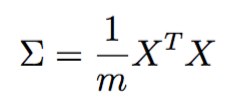```function [U, S] = pca(X)
%PCA Run principal component analysis on the dataset X
%   [U, S, X] = pca(X) computes eigenvectors of the covariance matrix of X
%   Returns the eigenvectors U, the eigenvalues (on diagonal) in S
%

% Useful values
[m, n] = size(X);

% You need to return the following variables correctly.
U = zeros(n);
S = zeros(n);

% ====================== YOUR CODE HERE ======================
% Instructions: You should first compute the covariance matrix. Then, you
%               should use the "svd" function to compute the eigenvectors
%               and eigenvalues of the covariance matrix.
%
% Note: When computing the covariance matrix, remember to divide by m (the
%       number of examples).
%

Sigma = X' * X / m;
[U, S, V] = svd(Sigma);

% =========================================================================

end```

```%% =============== Part 2: Principal Component Analysis ===============
%  You should now implement PCA, a dimension reduction technique. You
%  should complete the code in pca.m
%
fprintf('\nRunning PCA on example dataset.\n\n');

%  Before running PCA, it is important to first normalize X
[X_norm, mu, sigma] = featureNormalize(X);

%  Run PCA
[U, S] = pca(X_norm);

%  Compute mu, the mean of the each feature

%  Draw the eigenvectors centered at mean of data. These lines show the
%  directions of maximum variations in the dataset.
hold on;
drawLine(mu, mu + 1.5 * S(1,1) * U(:,1)', '-k', 'LineWidth', 2);
drawLine(mu, mu + 1.5 * S(2,2) * U(:,2)', '-k', 'LineWidth', 2);
hold off;

fprintf('Top eigenvector: \n');
fprintf(' U(:,1) = %f %f \n', U(1,1), U(2,1));
fprintf('\n(you should expect to see -0.707107 -0.707107)\n');

fprintf('Program paused. Press enter to continue.\n');
pause;```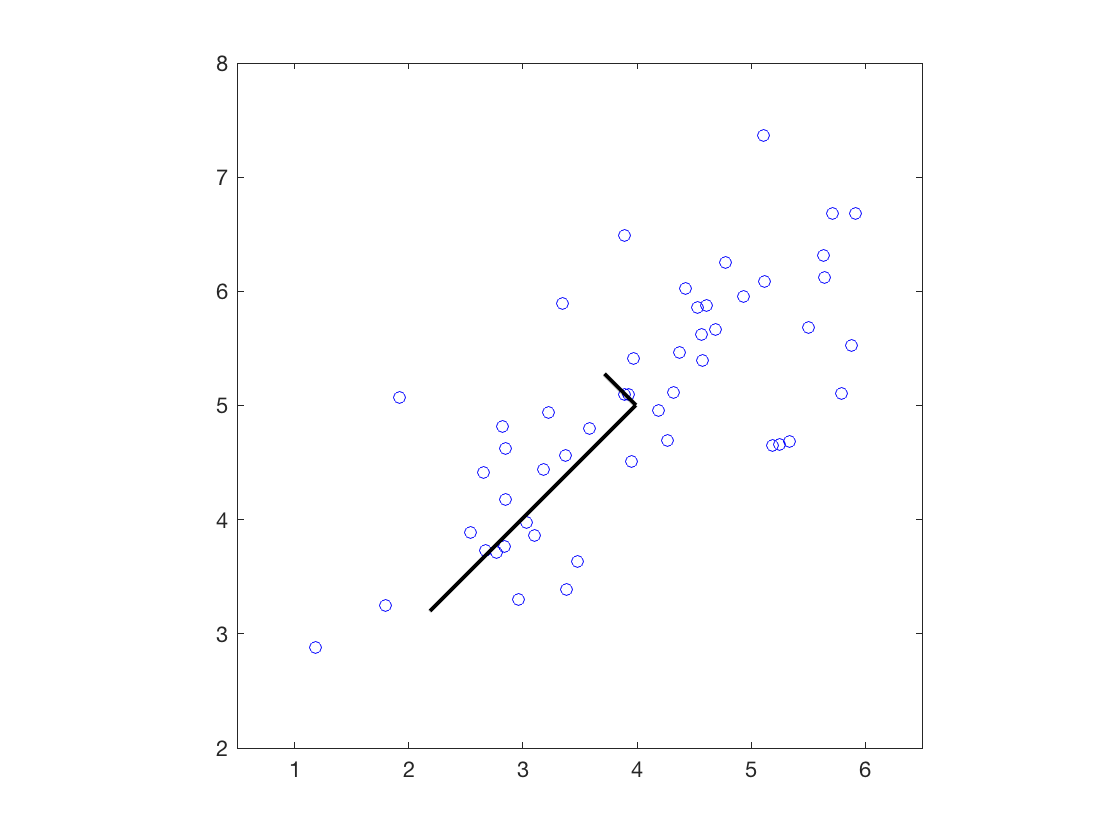### PCA降维

```function Z = projectData(X, U, K)
%PROJECTDATA Computes the reduced data representation when projecting only
%on to the top k eigenvectors
%   Z = projectData(X, U, K) computes the projection of
%   the normalized inputs X into the reduced dimensional space spanned by
%   the first K columns of U. It returns the projected examples in Z.
%

% You need to return the following variables correctly.
Z = zeros(size(X, 1), K);

% ====================== YOUR CODE HERE ======================
% Instructions: Compute the projection of the data using only the top K
%               eigenvectors in U (first K columns).
%               For the i-th example X(i,:), the projection on to the k-th
%               eigenvector is given as follows:
%                    x = X(i, :)';
%                    projection_k = x' * U(:, k);
%

x = X';
Z = x' * U(:, 1:K);

% =============================================================

end```

```%% =================== Part 3: Dimension Reduction ===================
%  You should now implement the projection step to map the data onto the
%  first k eigenvectors. The code will then plot the data in this reduced
%  dimensional space.  This will show you what the data looks like when
%  using only the corresponding eigenvectors to reconstruct it.
%
%  You should complete the code in projectData.m
%
fprintf('\nDimension reduction on example dataset.\n\n');

%  Plot the normalized dataset (returned from pca)
plot(X_norm(:, 1), X_norm(:, 2), 'bo');
axis([-4 3 -4 3]); axis square

%  Project the data onto K = 1 dimension
K = 1;
Z = projectData(X_norm, U, K);
fprintf('Projection of the first example: %f\n', Z(1));
fprintf('\n(this value should be about 1.481274)\n\n');```

### 还原数据

```function X_rec = recoverData(Z, U, K)
%RECOVERDATA Recovers an approximation of the original data when using the
%projected data
%   X_rec = RECOVERDATA(Z, U, K) recovers an approximation the
%   original data that has been reduced to K dimensions. It returns the
%   approximate reconstruction in X_rec.
%

% You need to return the following variables correctly.
X_rec = zeros(size(Z, 1), size(U, 1));

% ====================== YOUR CODE HERE ======================
% Instructions: Compute the approximation of the data by projecting back
%               onto the original space using the top K eigenvectors in U.
%
%               For the i-th example Z(i,:), the (approximate)
%               recovered data for dimension j is given as follows:
%                    v = Z(i, :)';
%                    recovered_j = v' * U(j, 1:K)';
%
%               Notice that U(j, 1:K) is a row vector.
%

v = Z';
X_rec = v' * U(:, 1:K)';

% =============================================================

end```

```X_rec  = recoverData(Z, U, K);
fprintf('Approximation of the first example: %f %f\n', X_rec(1, 1), X_rec(1, 2));
fprintf('\n(this value should be about  -1.047419 -1.047419)\n\n');

%  Draw lines connecting the projected points to the original points
hold on;
plot(X_rec(:, 1), X_rec(:, 2), 'ro');
for i = 1:size(X_norm, 1)
drawLine(X_norm(i,:), X_rec(i,:), '--k', 'LineWidth', 1);
end
hold off

fprintf('Program paused. Press enter to continue.\n');
pause;```## 人脸数据集### 主成分分析

```%% =========== Part 5: PCA on Face Data: Eigenfaces  ===================
%  Run PCA and visualize the eigenvectors which are in this case eigenfaces
%  We display the first 36 eigenfaces.
%
fprintf(['\nRunning PCA on face dataset.\n' ...
'(this mght take a minute or two ...)\n\n']);

%  Before running PCA, it is important to first normalize X by subtracting
%  the mean value from each feature
[X_norm, mu, sigma] = featureNormalize(X);

%  Run PCA
[U, S] = pca(X_norm);

%  Visualize the top 36 eigenvectors found
displayData(U(:, 1:36)');

fprintf('Program paused. Press enter to continue.\n');
pause;```### 降维与还原

```%% ============= Part 6: Dimension Reduction for Faces =================
%  Project images to the eigen space using the top k eigenvectors
%  If you are applying a machine learning algorithm
fprintf('\nDimension reduction for face dataset.\n\n');

K = 100;
Z = projectData(X_norm, U, K);

fprintf('The projected data Z has a size of: ')
fprintf('%d ', size(Z));

fprintf('\n\nProgram paused. Press enter to continue.\n');
pause;

%% ==== Part 7: Visualization of Faces after PCA Dimension Reduction ====
%  Project images to the eigen space using the top K eigen vectors and
%  visualize only using those K dimensions
%  Compare to the original input, which is also displayed

fprintf('\nVisualizing the projected (reduced dimension) faces.\n\n');

K = 100;
X_rec  = recoverData(Z, U, K);

% Display normalized data
subplot(1, 2, 1);
displayData(X_norm(1:100,:));
title('Original faces');
axis square;

% Display reconstructed data from only k eigenfaces
subplot(1, 2, 2);
displayData(X_rec(1:100,:));
title('Recovered faces');
axis square;

fprintf('Program paused. Press enter to continue.\n');
pause;```## 为了可视化进行的PCA

```%% === Part 8(a): Optional (ungraded) Exercise: PCA for Visualization ===
%  One useful application of PCA is to use it to visualize high-dimensional
%  data. In the last K-Means exercise you ran K-Means on 3-dimensional
%  pixel colors of an image. We first visualize this output in 3D, and then
%  apply PCA to obtain a visualization in 2D.

close all; close all; clc

% Re-load the image from the previous exercise and run K-Means on it
% For this to work, you need to complete the K-Means assignment first

% If imread does not work for you, you can try instead

A = A / 255;
img_size = size(A);
X = reshape(A, img_size(1) * img_size(2), 3);
K = 16;
max_iters = 10;
initial_centroids = kMeansInitCentroids(X, K);
[centroids, idx] = runkMeans(X, initial_centroids, max_iters);

%  Sample 1000 random indexes (since working with all the data is
%  too expensive. If you have a fast computer, you may increase this.
sel = floor(rand(1000, 1) * size(X, 1)) + 1;

%  Setup Color Palette
palette = hsv(K);
colors = palette(idx(sel), :);

%  Visualize the data and centroid memberships in 3D
figure;
scatter3(X(sel, 1), X(sel, 2), X(sel, 3), 10, colors);
title('Pixel dataset plotted in 3D. Color shows centroid memberships');

for i=1:2:360
%view(a,b):a????b????
view(i,20);
pause(0.06);
frame=getframe(gcf);
im=frame2im(frame);
[I,map]=rgb2ind(im,256);
if i==1
imwrite(I,map,'ex.gif','gif','Loopcount',Inf,'DelayTime',0.2);
else
imwrite(I,map,'ex.gif','gif','WriteMode','append','DelayTime',0.2);
end
end

fprintf('Program paused. Press enter to continue.\n');
pause;```### PCA降维

```%% === Part 8(b): Optional (ungraded) Exercise: PCA for Visualization ===
% Use PCA to project this cloud to 2D for visualization

% Subtract the mean to use PCA
[X_norm, mu, sigma] = featureNormalize(X);

% PCA and project the data to 2D
[U, S] = pca(X_norm);
Z = projectData(X_norm, U, 2);

% Plot in 2D
figure;
plotDataPoints(Z(sel, :), idx(sel), K);
title('Pixel dataset plotted in 2D, using PCA for dimensionality reduction');
fprintf('Program paused. Press enter to continue.\n');
pause;```### 评论 4

1. #4

笑脸是 : )

也是wo7年前 (2017-02-10)回复
2. #3

稍微简洁点

也是wo7年前 (2017-02-10)回复
3. #2

findClosestCentroids.m 我的实现是:
K = size(centroids, 1);
idx = zeros(size(X,1), 1);
[m, n] = size(X)
for i = 1 : m
X_tmp = ones(K, 1) * X(i,;
[val, idx(i, 1)]= min(sum((X_tmp – centroids).^2, 2), [], 1);
end

也是wo7年前 (2017-02-10)回复
4. #1

computeCentroids.m 我的实现是:
[m n] = size(X);
centroids = zeros(K, n);
for i = 1 : K
num = size(find(i == idx), 1);
centroids(i,= sum(X(find(i == idx),, 1) ./ num;
end

也是wo7年前 (2017-02-10)回复